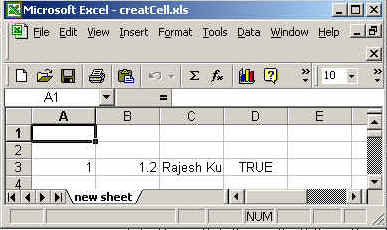Tutorials

# Create Excel Sheet Using JSP

In this program, we are going to create the cells and rows into excel sheet using java .

In this program, we are going to create the cells and rows into excel sheet using java .

# create excel sheet using jsp

In this program, we are going to create the cells and rows into excel sheet using java .You can create any number of cells and rows and also you can add values into cells.

The package we need to import is
java.io.*,
org.apache.poi.hssf.usermodel.HSSFSheet,
org.apache.poi.hssf.usermodel.HSSFCell,
org.apache.poi.hssf.usermodel.HSSFRow,
org.apache.poi.hssf.usermodel. HSSFWorkbook.

The org.apache.poi.hssf.usermodel.HSSFRow class is used to create a row  in which we can set the cells.

The org.apache.poi.hssf.usermodel.HSSFCell class is used to create a new cell and add the values into cells.

createRow((short)value):
This method is used to create  a new row. The argument "value" (short type) is the number for which we are going to create
the row e.g. createRow((short)0) is for creating 0th row.

createCell((short)value):
This method is used to create a new cell. The argument "value" (short type) is the number for which we are going to create the new cell  e.g. createCell((short)0) is for creating 0th cell.

setCellValue(values):
This method is used to add the value into the cell. You can add any data types into cell by using this method. In this example, we have used four data types int , float , string and boolean which are being added at 0th ,1st,2nd,3th  and 4th cells  into 0th row.

The code of the program is given below:

 ``` <%@ page import="org.apache.poi.hssf.usermodel.HSSFSheet"%> <%@ page import="org.apache.poi.hssf.usermodel.HSSFWorkbook"%> <%@ page import="org.apache.poi.hssf.usermodel.HSSFCell"%> <%@ page import="org.apache.poi.hssf.usermodel.HSSFRow"%> <%@ page import="java.io.*" %> <%try{  HSSFWorkbook wb = new HSSFWorkbook();  HSSFSheet sheet = wb.createSheet("new sheet");  HSSFRow row = sheet.createRow((short)0);  HSSFCell cell = row.createCell((short)0);  cell.setCellValue(1);  row.createCell((short)1).setCellValue(1.2);  row.createCell((short)2).setCellValue("Rajesh Kumar ");  row.createCell((short)3).setCellValue(true);   FileOutputStream fileOut = new FileOutputStream ("c:\\excel\\createCell.xls");   wb.write(fileOut);   fileOut.close();    }catch ( Exception ex ){    }  %>```

The output of the program is given below: